Related Articles

# What is the Difference between Interactive and Script Mode in Python Programming?

• Difficulty Level : Easy
• Last Updated : 05 Aug, 2021

Python is a programming language that lets you work quickly and integrate systems more efficiently. It is a widely-used general-purpose, high-level programming language. It was designed with an emphasis on code readability, and its syntax allows programmers to express their concepts in fewer lines of code. In the Python programming language, there are two ways in which we can run our code:

1. Interactive mode

Attention reader! All those who say programming isn't for kids, just haven't met the right mentors yet. Join the  Demo Class for First Step to Coding Coursespecifically designed for students of class 8 to 12.

The students will get to learn more about the world of programming in these free classes which will definitely help them in making a wise career choice in the future.

2. Script mode

In this article, we’ll get to know what these modes are and how they differ from each other.

### Interactive mode

Interactive etymologically means “working simultaneously and creating impact of our work on the other’s work”. Interactive mode is based on this ideology only. In the interactive mode as we enter a command and press enter, the very next step we get the output. The output of the code in the interactive mode is influenced by the last command we give. Interactive mode is very convenient for writing very short lines of code. In python is it also known as REPL which stands for Read Evaluate Print Loop. Here, the read function reads the input from the user and stores it in memory. Eval function evaluates the input to get the desired output. Print function outputs the evaluated result. The loop function executes the loop during the execution of the entire program and terminates when our program ends. This mode is very suitable for beginners in programming as it helps them evaluate their code line by line and understand the execution of code well.

How to run python code in Interactive mode?

In order to run our program in the interactive mode, we can use command prompt in windows, terminal in Linux, and macOS. Let us see understand the execution of python code in the command prompt with the help of an example:

Example 1:

To run python in command prompt type “python”.  Then simply type the Python statement on >>> prompt. As we type and press enter we can see the output in the very next line.

## Python3

 `# Python program to display "Hello GFG"``print``(``"Hello GFG"``)`

Output: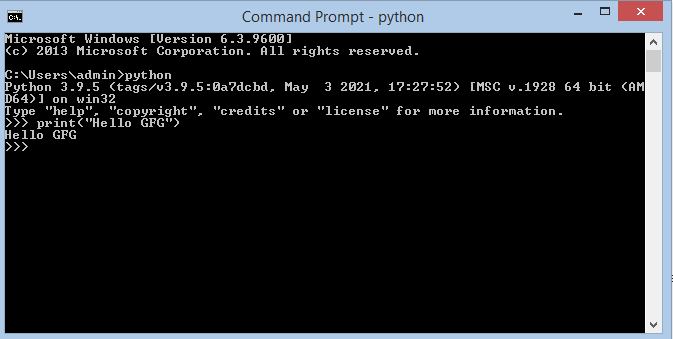Example 2

Let us take another example in which we need to perform addition on two numbers and we want to get its output. We will declare two variables a and b and store the result in a third variable c. We further print c. All this is done in the command prompt.

## Python3

 `# Python program to add two numbers``a ``=` `2` `b ``=` `3`` ` `# Adding a and b and storing result in c``c ``=` `a ``+` `b `` ` `# Printing value of c``print``(c) `

Output: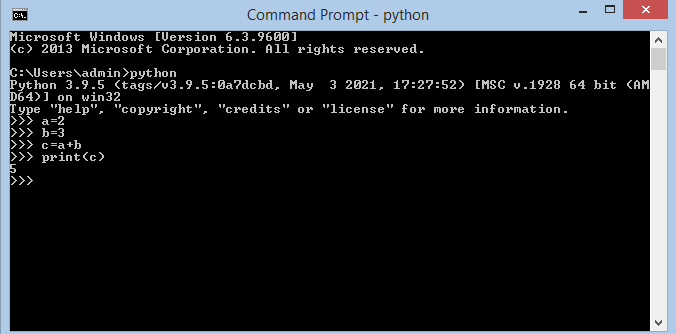We can see the desired output on the screen. This kind of program is a very short program and can be easily executed in interactive mode.

Example 3:

In this example, we will multiply two numbers and take the numbers as an input for two users. You will see that when you execute the input command, you need to give input in the very next line, i.e. code is interpreted line by line.

## Python3

 `# Python program to take input from user`` ` `# Taking input from user``a ``=` `int``(``input``()) `` ` `# Taking input from user``b ``=` `int``(``input``()) `` ` `# Multiplying and storing result``c ``=` `a ``*` `b `` ` `# Printing the result``print``(c) `

Output: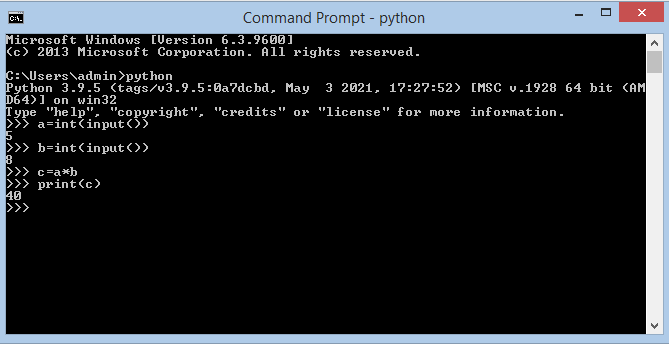• The interactive mode is not suitable for large programs.
• The interactive mode doesn’t save the statements. Once we make a program it is for that time itself, we cannot use it in the future. In order to use it in the future, we need to retype all the statements.
• Editing the code written in interactive mode is a tedious task. We need to revisit all our previous commands and if still, we could not edit we need to type everything again.

### Script Mode

Script etymologically means a system of writing. In the script mode, a python program can be written in a file. This file can then be saved and executed using the command prompt. We can view the code at any time by opening the file and editing becomes quite easy as we can open and view the entire code as many times as we want. Script mode is very suitable for writing long pieces of code. It is much preferred over interactive mode by experts in the program. The file made in the script made is by default saved in the Python installation folder and the extension to save a python file is “.py”.

How to run python code in script mode?

In order to run a code in script mode follow the following steps.

Step 1: Make a file using a text editor. You can use any text editor of your choice(Here I use notepad).

Step 2: After writing the code save the file using “.py” extension.

Step 3: Now open the command prompt and command directory to the one where your file is stored.

Step 4: Type python “filename.py” and press enter.

Step 5: You will see the output on your command prompt.

Let us understand these steps with the help of the examples:

Example 1:

In order to execute “Hello gfg” using script mode we first make a file and save it.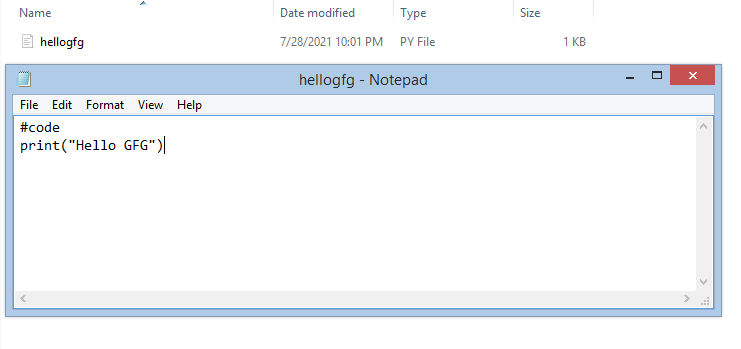Now we use the command prompt to execute this file.

Output: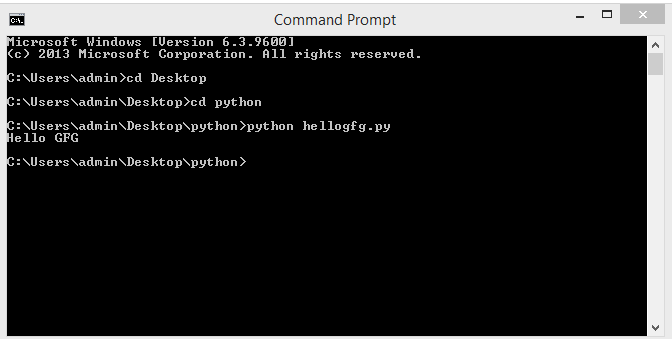Example 2:

Our second example is the same addition of two numbers as we saw in the interactive mode. But in this case, we first make a file and write the entire code in that file. We then save it and execute it using the command prompt.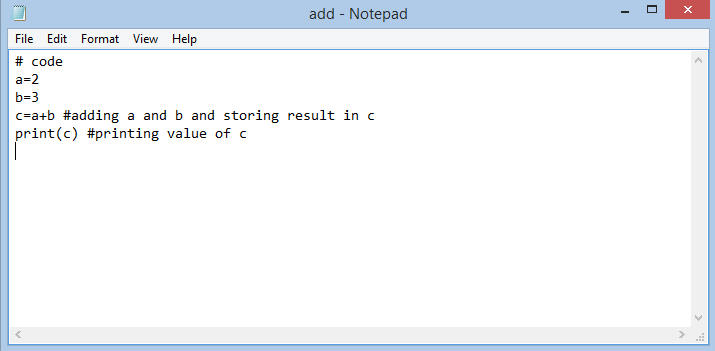Output: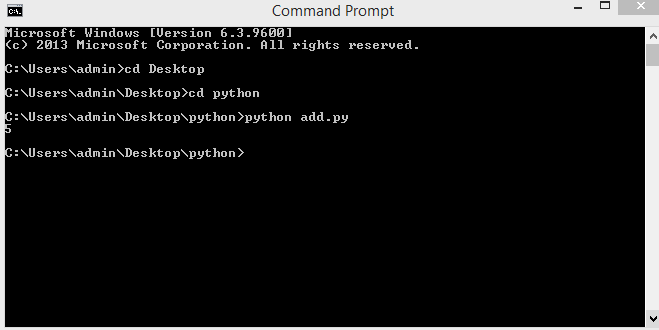Example 3:

In this example, we write the code for multiplying two numbers. And the numbers which are to be multiplied are taken by the user as an input. In the interactive mode, we saw that as we write the command so does it asks for the input in the very next line. But in script mode we first code the entire program save and then run it in command prompt. The python interpreter executes the code line by line and gives us the result accordingly.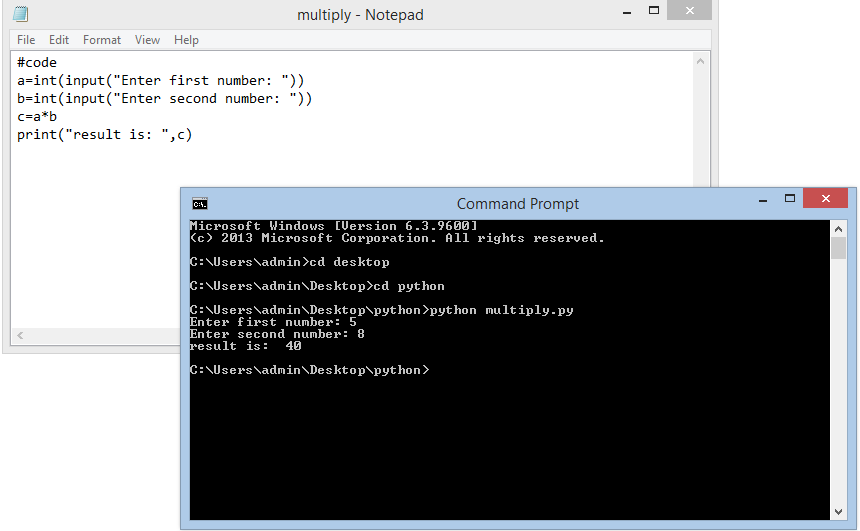In this example, we see that the whole program is compiled and the code is executed line by line. The output on the shell is entirely different from the interactive mode.

### Difference between Interactive mode and Script mode

My Personal Notes arrow_drop_up